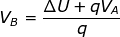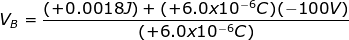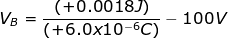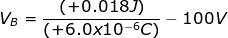# Potential Energy: Electric Potential Formula

Potential Energy: Electric Potential Formula

Potential energy is energy that is stored in a system, based on the position of objects. A charged particle in an electric field has potential energy because of the electrostatic force that can act on it. It is often useful to be able to describe the potential energy per unit charge at a certain position. This potential energy per unit charge is called electric potential (or simply "potential"). Like all work and energy, the unit of potential energy is the Joule (J), where 1 J = 1 kg∙m2/s2 . The unit of charge is the Coulomb (C), and the unit of electric potential is the Volt (V), which is equal to a Joule per Coulomb (J/C).

potential energy = (charge of particle)(electric potential)

U = qV

U = potential energy, with units J (Joules)

q = the charge of the point particle, with units C (Coulombs)

V = an electric potential, with units V = J/C (Volts, equal to Joules per Coulomb)

Potential Energy: Electric Potential Formula Questions:

1) A point particle has a charge of -8.0 μC. It moves from point A, with electric potential VA = +200 V, to point B, with electric potential VB = +600 V. What is the change in potential energy as a result of this movement?

Answer: The change in potential energy, ∆U, can be found using the formula:

∆U = UB - UA

∆U = qVB - qVA

∆U = (-8.0 μC)( +600 V) - (-8.0 μC)(+200 V)

The charge is given in terms of micro-Coulombs (μC): 1.0 μC = 1.0 x 10-6C. The charge needs to be converted to the correct units before solving the equation:

∆U = (-8.0 x 10-6C)(+600 J/C)-(-8.0 x 10-6C)(+200 J/C)

∆U = -0.0032 J

The change in potential energy due to the movement of the point particle is -0.0032 J.

2) A point particle has a charge of +6.0 μC. It moves from point A, with electric potential VA = -100 V, to point B. In the process, the potential energy changes by +0.0018 J. What is the electric potential at point B?

Answer: The electric potential can be found by rearranging the formula:

∆U = UB - UAThe charge is given in terms of micro-Coulombs (μC): 1.0 μC = 1.0 x 10-6C. The charge needs to be converted to the correct units before solving the equation:VB = 300 V - 100 V

VB = +200 V

The electric potential at position B is +200 V.